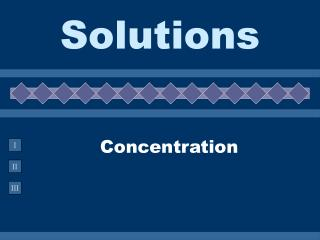Download PresentationConcentration

# Concentration - PowerPoint PPT PresentationDownload Presentation## Concentration

- - - - - - - - - - - - - - - - - - - - - - - - - - - E N D - - - - - - - - - - - - - - - - - - - - - - - - - - -
##### Presentation Transcript

1. Solutions Concentration

2. Concentration Units • The amount of solute in a solution. • Describing Concentration • % by mass - medicated creams • % by volume - rubbing alcohol • ppm, ppb - water contaminants • molarity - used by chemists • molality - used by chemists

3. moles solute = Molarity ( M ) liters of solution Molarity

4. PROBLEM: Dissolve 5.00 g of NiCl2•6 H2O (237.7 g/mol) in enough water to make 250 mL of solution. Calculate the Molarity. Step 1: Calculate moles of NiCl2•6H2O Step 2: Calculate Molarity [NiCl2•6 H2O = 0.0841 M

5. What mass of oxalic acid, H2C2O4, is required to make 250. mL of a 0.0500M solution? Step 1: Change mL to L:250 mL * 1L/1000mL = 0.250 L Step 2: Calculate Moles = (0.0500 mol/L) (0.250 L) = 0.0125 moles Step 3: Convert moles to grams. (0.0125 mol)(90.00 g/mol) = 1.13 g

6. Learning Check How many grams of NaOH are required to prepare 400. mL of 3.0 M NaOH solution? 1) 12 g 2) 48 g 3) 300 g

7. mol solute m of solution = kilograms solvent Two Other Concentration Units MOLALITY, m % by mass grams solute grams solution % by mass =

8. Percent Composition • This is the mass of the solute divided by the mass of the solution (mass of solute plus mass of solvent), multiplied by 100. • Example: Determine the percent composition by mass of a 100 g salt solution which contains 20 g salt. (20 g NaCl / 100 g solution) x 100 = 20% NaCl solution

9. mass of solvent only 1 kg water = 1 L water Molality

10. Calculating Concentrations Dissolve 62.1 g (1.00 mol) of ethylene glycol in 250. g of H2O. Calculate m & % of ethylene glycol (by mass). Calculate molality Calculate weight %

11. Molality • Find the molality of a solution containing 75 g of MgCl2 in 250 mL of water. 75 g MgCl2 1 mol MgCl2 95.21 g MgCl2 0.25 kg water = 3.2m MgCl2

12. Molality • How many grams of NaCl are req’d to make a 1.54m solution using 0.500 kg of water? 0.500 kg water 1.54 mol NaCl 1 kg water 58.44 g NaCl 1 mol NaCl = 45.0 g NaCl

13. Dilutions • Preparation of a desired solution by adding water to a concentrate. • Moles of solute remain the same.

14. Dilution • What volume of 15.8M HNO3 is required to make 250 mL of a 6.0M solution? GIVEN: M1 = 15.8M V1 = ? M2 = 6.0M V2 = 250 mL = 0.250 L WORK: M1 V1 = M2 V2 (15.8M)V1 = (6.0M)(0.250 L) V1 = 0.095 L of 15.8M HNO3